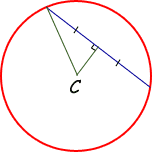SEARCH HOMEMath Central Quandaries & QueriesQuestion from venus, a student: a chord of 48mm long is 7mm from the center of the circle. What is the radius of the circle?Hi Venus.

The perpendicular bisector of a chord will always pass through the center of the circle. This is the distance of the chord from the center.Can you see the right triangle implied by this? What does Pythagoras' Theorem tell you about the relationship of the sides of the right triangle?

Cheers,
Stephen La Rocque.Math Central is supported by the University of Regina and The Pacific Institute for the Mathematical Sciences.